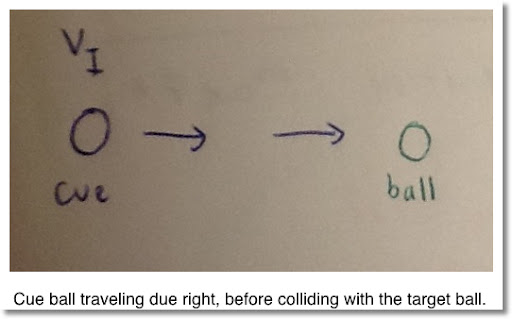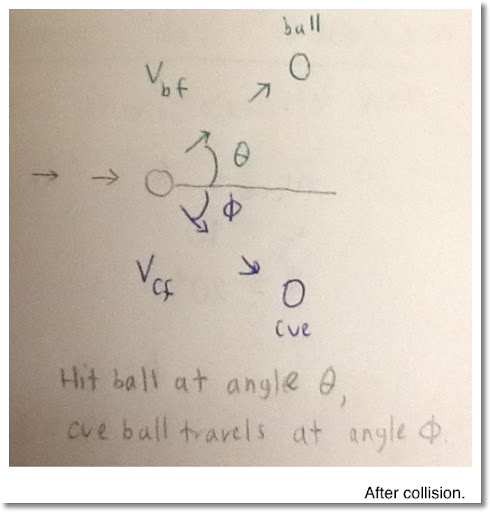## Saturday, March 17, 2012

### Billiards - Determining Final Velocity (Program for TI 84+ and HP 50g too!)

Welcome to blog entry #64!

HAPPY ST PATRICK'S DAY (WEEKEND)!

Billiards and Math

Ever wondered what happens with you take the cue stick, strike the cue ball, hit your target ball and the target ball sails right into a pocket? If you played billiards, commonly known as pool, you have experienced this many times.

We can use mathematics and physics to describe what happens when the cue ball hits the target ball. Since the balls do not "stick" together like steamed rice, the collision is considered to be nearly elastic.

In an elastic collision, two properties are conserved: momentum and kinetic energy. Momentum is the product of mass and it's velocity. Kinetic energy is the energy of movement. The equations for momentum and kinetic energy are, respectively:

P = m * v

KE = 1/2 * m * v^2

Note that direction is not considered in calculating kinetic energy.

Problem:

You hit a cue ball at a certain velocity VI, and hit a target ball at angle θ. We want to find out the direction of the cue ball after collision and the velocity of both balls.

Assume the following:

1. The masses of the cue ball and the target ball are the same. This will allow a great simplicafication in calculation, as we will see.

2. The Intial direction of the ball is 0° (due right). As a visual, you are on the left hitting the ball to due right.

Note the diagrams below:3. The angles created after the hit add up to 90°.

From the diagram above, θ + Φ = 90°.

4. The cue ball does not hit any balls other than the target ball. If more than two balls were involved, calculation can get hairy, quick.

5. The cue ball is hit at it's sweet spot, which eliminates any friction developing between the ball and the felt of the pool table. According to The Real World Physics Problems web site, the sweet spot is 1.4 times the ball's radius.

An American billiard ball has a diameter of about 2.25 in, or 5.715 cm. The ball has a typical weight of 5.5 to 6 oz, or 156 to 170 grams. This link has more information on billiard balls.

Solution, Assuming All Masses Are Equal

Let:

VI = initial velocity of the cue ball, going due right. In the x-direction, VI cos 0° = VI. In the y-direction, VI sin 0° = 0, as expected.

VCF = final velocity of the cue ball

VBF = final velocity of the target ball. Note since the target ball was at rest, it's initial velocity is zero.

Φ = Angle where the cue ball goes after collision. Simply put, Φ = 90° - θ.

MC = mass of the cue ball

MB = mass of the target ball

Note: We assumed the masses were equal, MB = MC.

Momentum and kinetic energy has to be preserved. So for momentum in the x-direction:

MC * VI = MB * VBF cos θ + MC * VCF cos Φ

and momentum in the y-direction:

0 = MB * VBF sin θ - MC * VCF sin Φ

Note: Φ is a angle in the clockwise direction and sin (-Φ) = -sin Φ

and for kinetic energy:

1/2 * MC * VI^2 = 1/2 * MB * VBF^2 + 1/2 * MC * VCF^2

Note: Since mass is the same for all balls (MC = MB), mass "cancels" out in all three equations.

To Recap:
VI = VBF cos θ + VCF cos Φ
0 = VBF sin θ - VCF sin Φ
1/2 * VI^2 = 1/2 * VBF^2 + 1/2 * VCF^2

Making use of the trigonometric identities:

sin (90° - x) = cos x
cos (90° - x) = sin x

The equations simplify to:
VI = VBF cos θ + VCF sin θ
0 = VBF sin θ - VCF cos θ
1/2 * VI^2 = 1/2 * VBF^2 + 1/2 * VCF^2

Observe that if we solve for VBF in the second equation, we get:
VBF = VCF * (cos θ / sin θ)

Then:
VI = VBF cos θ + VCF sin θ
VI = VCF * (cos θ / sin θ) *cos θ + VCF sin θ
VI = VCF * (cos^2 θ / sin θ ) + VCF sin θ
VI = (VCF * cos^2 θ + VCF sin^2 θ) / sin θ

Note: sin^2 x + cos^2 x = 1 for an angle x.

And:
VI = VCF / sin θ

Solving for VCF yields:
VCF = VI sin θ

Let's get VBF in terms of VI:
VBF = VCF * (cos θ / sin θ)
VBF = VI sin θ* (cos θ / sin θ)
VBF = VI cos θ

Before we can celebrate, we need to satisfy the conservation of kinetic energy, not just momentum.
1/2 * VI^2 = 1/2 * VBF^2 + 1/2 * VCF^2

First, let's just multiply everything by 2:
VI^2 = VBF^2 + VCF^2
VI^2 = (VI cos θ )^2 + (VI sin θ)^2
VI^2 = VI^2 * (cos^2 θ + sin^2 θ )
VI = VI

So this checks out because we assumed the masses of both the cue ball and target ball were equal. .

Final solution - All Masses being Equal:

VBF = VI cos θ
VCF = VI sin θ
Φ = 90° - θ

What if the Mass of the Cue Ball and Target Ball are not equal?

Let:

MC = mass of the cue ball

MB = mass of the target ball

If I use the strategy of solving for VCF and VBF above, I get:

VCF = VI * sin θ.
VBF = (MC / MB) * VI * cos θ

We are working with elastic collisions, so conservation of kinetic energy must be considered:

1/2 * MC * VI^2 = 1/2 * MB * VBF^2 + 1/2 * MC * VCF^2

But:
1/2 * MB * VBF^2 + 1/2 * MC * VCF^2
= 1/2 * (MB * (VI * cos θ * MC / MB)^2 + MC * VI^2 * sin^2 θ))
= 1/2 * MC * VI^2 * (cos^2 θ / MB + sin^2 θ)
≠ 1/2 * MC * VI^2

Yep. No go. Confession, I thought the above way was good to go when MC ≠ MB too.

Here is a real solution if MC ≠ MB:

VBF = (MC * VCF) / (MB * tan θ)

And we have to solve the quadratic equation for VCF:

0 = VCF^2 * (MB * MC^2 * sin θ + MC^2 * cos^2 θ)
+ VCF * (MB * MC)
+ (MB * sin θ * (MC * VI + MC * VI^2))

The following calculator programs solve for Φ, VCF, and VBF with the assumption that the mass of both the cue ball and target ball are the same.

Variables for the programs:
C = VI
A = θ

TI 84 Plus:

NAME: BILLIARD

Degree
Disp "Deg MODE"
Input "INIT. VEL.",C
Input "ANGLE HIT",A
C*SIN(A) → S
C*COS(A) → T
Disp "ANGLE CUE=", 90 - A
Pause
Disp "FINAL VEL. CUE=",S
Disp "FINAL VEL. BALL=",T

HP 50g:

NAME: BILLIARD

<< DEG
"Degrees Mode" MSGBOX
"Initial Velocity, Cue" PROMPT
"Hit Angle" PROMPT
→ C A
<< "Finals:"
90 A - →NUM "Angle, Cue" →TAG
C A SIN * →NUM "Vel., Cue" →TAG
C A COS * →NUM "Vel., Ball" →TAG
>> >>

Test data:
VI = 5, θ = 15°; Results: VCF ≈ 1.2941, VBF ≈ 4.8296

VI = 2, θ = 30°; Results: VCF = 1, VBF = √3 ≈ 1.7321

Thank you, hope you enjoyed this blog entry. Next time I probably be blogging on a third generation iPad. For the first 64 entries, I have been using the Blogger+ App by ThinkTek Co. Ltd. on my iPad 2. Thanks everyone!

Eddie

This blog is property of Edward Shore. © 2012

### Retro Review: Radio Shack EC-4026

Retro Review:   Radio Shack EC-4026 Quick Facts: Model:  EC-4026 Company:  Radio Shack Equivalent Of:  Casio fx-4500P, Casio fx-4500PA Years...